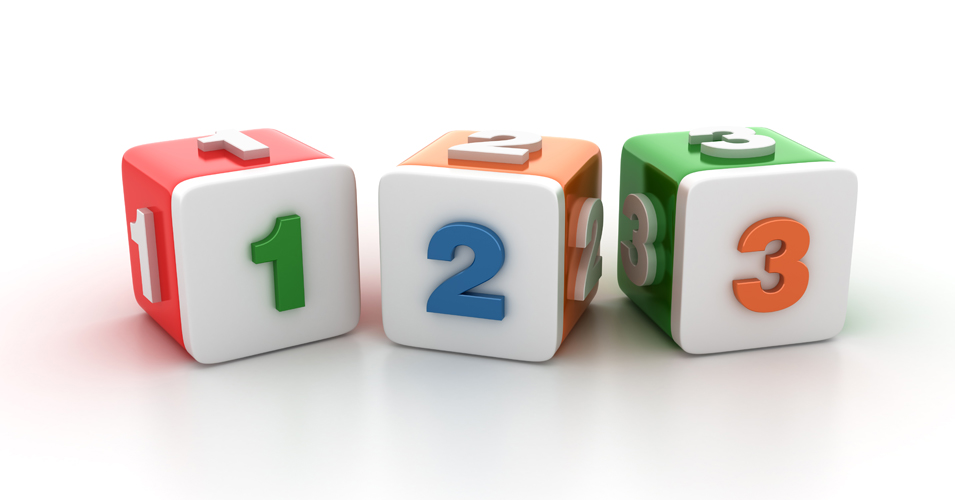• Save

# Number System Questions for Competitive Exams

10 months ago 5.7K ViewsThe number system is one of the most important sections included in the aptitude section because the topic is easier for the students in general but its harder actually. There are many systems for representing numbers, but the most prevalent system in these systems is the number system which also known as the Dashamic system / Hindu-Arabic numeration system. Under this system, we use signs (0, 1, 2, 3, 4, 5, 6, 7, 8, and 9) to show a number which is called a digit. The same ten digits we use to show a number. Also, the Competition Exam covers 3 to 4 questions related to the number system under the Aptitude section.

So here today through this blog, I am providing you important questions based on the number system, which questions are often asked in all competitive exams like Bank Clerk, Bank PO, Railways, SSC, UPSC. Apart from this, questions related to the number system can be solved easily by knowledge of the required rules and everyday practice, so let's know -

You can download the  Application(IOS and Android) for Start Practice with Test Series and  Practice Tests here.

## Number System Questions

Q :

When n is divided by 6, the remainder is 4. When 2n is divided by 6, the remainder is :

(A) 4

(B) 2

(C) 0

(D) 1

Correct Answer : B

Q :

A number x when divided by 289 leaves 18 as the remainder. The same number when divided by 17 leaves y as a remainder. The value of y is

(A) 3

(B) 1

(C) 5

(D) 2

Correct Answer : B

Q :

A, B, C and D purchase a gift worth Rs. 60. A pays $${1\over2}$$ of what others are paying. B pays $${1\over 3}$$ of  what others are paying and C  pays $${1\over 4}$$ of what others are  paying. What is the amount paid by D?

(A) 12

(B) 14

(C) 13

(D) 15

Correct Answer : C

Q :

In an office, there are 108 tables and 132 chairs. If $${1\over6}$$ of the tables and $${1\over4}$$ of the chairs are broken. How many people can work in the office if each person requires one table and one chair?

(A) 92

(B) 99

(C) 86

(D) 90

Correct Answer : D

Q :

The difference of a number consisting of two digits from the number formed by inter changing the digits is always divisible by:

(A) 11

(B) 6

(C) 10

(D) 9

Correct Answer : D

Q :

The total number of integers between 100 and 200, which are divisible by both 9 and 6, is

(A) 7

(B) 8

(C) 5

(D) 6

Correct Answer : D

Q :

The least number, which must be added to 6709 to make it exactly divisible by 9, is

(A) 7

(B) 2

(C) 5

(D) 4

Correct Answer : C

Q :

In a two digit number if it is known that its unit digit exceeds its tens digit by 2 and that the product of the given number and the sum of its digits is equal to 144, then the number is

(A) 26

(B) 24

(C) 46

(D) 42

Correct Answer : B

Q :

0.1 and   $${5\over 8}$$ of a bamboo are in mud and water respectively and the rest of length 2.75 m is above water. What is the length of the bamboo?

(A) 27.5 m

(B) 20 m

(C) 10 m

(D) 30 m

Correct Answer : C

Q :

461 + 462 + 463 + 464 is divisible by:

(A) 3

(B) 13

(C) 10

(D) 11

Correct Answer : C

## About author

### Vikram Singh

Providing knowledgable questions of Reasoning and Aptitude for the competitive exams.

## Report Error: Number System Questions for Competitive Exams

Please Enter Message
Error Reported Successfully